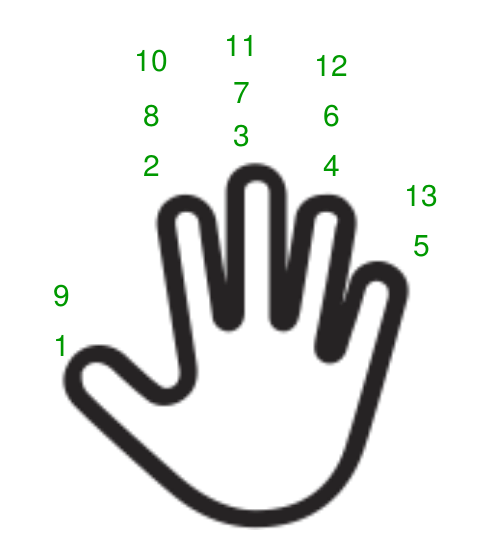Related Articles

# Program to Count numbers on fingers

• Difficulty Level : Easy
• Last Updated : 15 Jun, 2021

Count the given numbers on your fingers and find the correct finger on which the number ends.

• The first number starts from the thumb, second on the index finger, third on the middle finger, fourth on the ring finger, and fifth on the little finger.
• Again six comes on the ring finger and so on.
• Here we observer a pattern, 8(last number) and 2 ends up in 2nd position, 3rd or 7th on the middle finger, and so on.
• The pattern keeps repeating after every 8 numbers
• 1 to 8
• 9 to 16
• 17 to 24, and so onExamples:

```Input : 17
Output :1

Input :27
Output :3```

## C++

 `// CPP Program to Count numbers on fingers``#include ``using` `namespace` `std;` `int` `count_num_finger(``int` `n)``{``    ``int` `r = n % 8;``    ``if` `(r == 0)``        ``return` `2;``    ``if` `(r < 5)``        ``return` `r;``    ``else``        ``return` `10 - r;``}` `// Driver code``int` `main()``{``    ``int` `n;``    ``n = 30;``    ``cout << count_num_finger(n);` `    ``return` `0;``}`

## Java

 `// Java Program to Count numbers on fingers``class` `GFG``{``static` `int` `count_num_finger(``int` `n)``{``    ``int` `r = n % ``8``;``    ``if` `(r == ``0``)``        ``return` `2``;``    ``if` `(r < ``5``)``        ``return` `r;``    ``else``        ``return` `10` `- r;``}` `// Driver Code``public` `static` `void` `main(String[] args)``{``    ``int` `n;``    ``n = ``30``;``    ``System.out.println(count_num_finger(n));``}``}` `// This code is contributed``// by Mukul Singh`

## Python3

 `def` `count_num_finger( n ):``    ``r ``=` `n ``%` `8``    ``if` `r ``=``=` `0``:``        ``return` `2``    ``if` `r < ``5``:``        ``return` `r``    ``else``:``        ``return` `10` `-` `r` `# Driver Code``n ``=` `30``print``(count_num_finger(n))` `# This code is contributed by "Sharad_Bhardwaj".`

## C#

 `// C# Program to Count numbers on fingers``using` `System;``    ` `class` `GFG``{``    ` `static` `int` `count_num_finger(``int` `n)``{``    ``int` `r = n % 8;``    ``if` `(r == 0)``        ``return` `2;``    ``if` `(r < 5)``        ``return` `r;``    ``else``        ``return` `10 - r;``}` `// Driver Code``public` `static` `void` `Main(String[] args)``{``    ``int` `n;``    ``n = 30;``    ``Console.WriteLine(count_num_finger(n));``}``}` `// This code is contributed by Princi Singh`

## PHP

 ``

## Javascript

 ``

Output:

`4`

Asked in Paytm Campus Placement August 2017
This article is contributed by Dinesh Malav. If you like GeeksforGeeks and would like to contribute, you can also write an article using write.geeksforgeeks.org or mail your article to review-team@geeksforgeeks.org. See your article appearing on the GeeksforGeeks main page and help other Geeks.